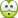# Homogeneous Differential Equation

## Homework Statement

Solve the following differential equation:
y' = y / [ x + √(y^2 - xy)]

2. The attempt at a solution
Using the standard method for solving homogeneous equations, setting u = y/x, I arrive at the following:

± dx/x = [1±√(u^2-u) ]/ [u√(u^2-u)] which in turn, I get the following integral after simplifying:

∫du/[u√(u^2-u)], seems quite unsolvable to me...

kuruman
Homework Helper
Gold Member
Note that$$\frac{1}{u\sqrt{u^2-u}}=\frac{1}{u^{3/2}(u-1)^{1/2}}$$Here's where the guessing and experience with derivatives comes in. The idea is to write this as a perfect differential which would make the integration trivial. You know that the term ##u^{3/2}## in the denominator arises by taking the derivative of ##1/u^{1/2}## and the term ##(u-1)^{1/2}## in the denominator arises by taking the derivative of ##(u-1)^{1/2}##. You also know the product rule of differentiation. What do you get when you take the derivative of ##\frac{(u-1)^{1/2}}{u^{1/2}}~##?

lurflurf
Homework Helper
Well , according to wolfram the integral $$\int \frac{du}{u\sqrt{u^2-u}}=2\sqrt{\frac{u-1}{u}}$$
$$\int \frac{du}{u\sqrt{u^2-u}}=\int \frac{du}{u^2\sqrt{1-1/u}}=\int \frac{d(1-1/u)}{\sqrt{1-1/u}}=\int 2d\sqrt{1-1/u}$$

•vela, MathematicalPhysicist and Delta2
Woah alright, great...Ray Vickson
Homework Helper
Dearly Missed
$$\int \frac{du}{u\sqrt{u^2-u}}=\int \frac{du}{u^2\sqrt{1-1/u}}=\int \frac{d(1-1/u)}{\sqrt{1-1/u}}=\int 2d\sqrt{1-1/u}$$

This is not quite right; it should be
$$\int \frac{d\:\text{anything}}{\sqrt{\text{anything}} }= 2 \sqrt{ \text{anything}}$$

Delta2
Homework Helper
Gold Member
This is not quite right; it should be
$$\int \frac{d\:\text{anything}}{\sqrt{\text{anything}} }= 2 \sqrt{ \text{anything}}$$
I don't understand where you disagree, isn't it ##\frac{d f}{\sqrt{f}}=2d(\sqrt{f)}## and then the integral operator cancels out with the differential operator d and leaves just ##2\sqrt{f}##

Ray Vickson